# COMBINATION OF RESISTORS

## Electricity of Class 9

Many times we have to join two or more resistances to get the desirable resistance. There are two ways in which resistances be joined:

• Resistances in series
• Resistances in Parallel

## RESISTANCES IN SERIES

A number of resistances are said to be connected in series if they are joined end to end and the same current flows through each one of them, when a potential difference is applied across the combination.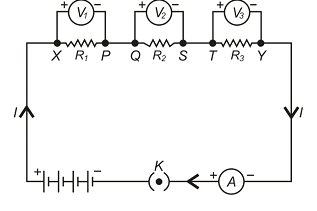R1, R2, R3 – Resistances in series.

V – Total potential difference across XY.

V1, V2, V3 – Potential difference across R1, R2, R3 respectively.

I – Current flowing through combination.

So, V = V1 + V2 + V3 ... (i) [Potential difference gets divided among  resistances joined in series]

According to Ohm's Law:

V1 = IR1 ... (ii)

V2 = IR2 ... (iii)

V3 = IR3 ... (iv)

Let R is the resultant or equivalent resistance of the combination. Then

V = IR ... (v)

From (i), (ii), (iii), (iv) and (v) we get that:

IR = IR1 + IR2 + IR3

IR = I(R1 + R2 + R3)

∴ R = R1 + R2 + R3

### THINGS TO REMEMBER IN SERIES CONNECTION:

• When a number of resistances are connected in series, the equivalent or resultant resistance is equal to the sum of individual resistances and resultant resistance is greater than any individual resistance.
• If n resistances each of value R are connected in series, the equivalent resistance Re is given by:

Re = R + R + R .......... n times

Re = nR

Re = Number of resistors × resistance of each resistor

• Equal current flows through each resistance and it is also equal to the total current in the circuit. This is because there is no other path along which the current can flow.
• The potential difference across the ends of the combination is distributed across the ends of each of the resistances. The potential difference across any one of the resistances is directly proportional to its resistance.
• The equivalent resistance when used in place of the combination of resistances produces the same current with the same potential difference applied across its ends.
• When two or more resistances are joined in series, the result is the same as increasing the length of the conductor. In both cases the resultant resistance is higher.
• In a series combination, the equivalent resistance is greater than the greatest resistance in the combination.

### RESISTANCES IN PARALLEL:

A number of resistors are said to be in a parallel connection if one end of each resistance is connected to one point and the other is connected to another point. The potential difference across each resistor is the same and is equal to the applied potential difference between the two points.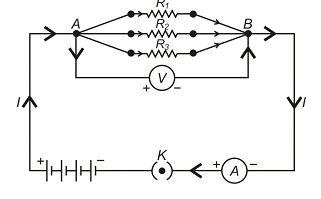R1, R2, R3 – Three resistances in parallel connection.

V – Potential difference across A and B.

I – Total current flowing between A and B.

I1, I2, I3 – Current flowing through R1, R2, R3 respectively.

I = I1 + I2 + I3 ... (i) [In parallel connection, the current gets divided among the resistances]

The potential difference across R1, R2 and R3 is same, therefore, according to Ohm's law: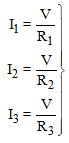... (ii)

Let Re be the equivalent resistance. Thus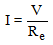... (iii)

From equation (i), (ii) and (iii) we get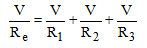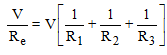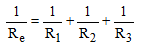### THINGS TO REMEMBER IN PARALLEL CONNECTION:

• When a number of resistances are connected in parallel, the reciprocal of the equivalent or resultant resistance is equal to the sum of reciprocals of the individual resistances and is always smaller than the individual resistances. This is because there are a number of paths for the flow of electrons.
• If there are n resistances connected in parallel and each resistance has a value of R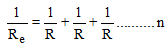times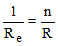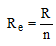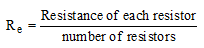• The potential difference across each resistance is the same and is equal to the total potential difference across the combination.
• The total current divides itself and different current flows through each resistor. The maximum current flows through the resistor having minimum resistance and vice versa.
• If an equivalent resistance Re is connected in place of combination, it produces the same current for the same potential difference applied across its ends.
• In a parallel combination, the equivalent resistance is lesser than the least of all the resistances.
• If two resistances R1 and R2 are connected in parallel then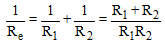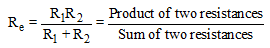•  If there are n resistors each of resistance R – Let RS be the resultant resistance of series combination and Rp be the resultant resistance of parallel combination.

Then, RS = nR

Rp =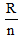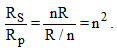.

### HEATING EFFECT OF ELECTRIC CURRENT:

Energy exists in various forms such as mechanical energy, heat energy, chemical energy, electrical energy, light energy and nuclear energy. According to the law of conservation of energy, energy can be transformed from one form to another.

In our daily life we use many devices where the electrical energy is converted into heat energy, light energy, chemical energy or mechanical energy. When an electric current is passed through a metallic wire like filament of an electric heater, oven or water heater, the filament gets heated up and here electrical energy is converted into heat energy. This is known as 'heating effect of current'.

It is a matter of common experience that a wire gets heated up when electric current flows through it. Why does this happen? A metallic conductor has a large number of free electrons in it. When a potential difference is applied across the ends of a metallic wire, the free electrons begin to drift from the low potential to the high potential region. These electrons collide with the positive ions (the atoms which have lost their electrons). In these collisions, energy of the electrons is transferred to the positive ions and they begin to vibrate more violently. As a result, heat is produced. Greater the number of electrons flowing per second, greater will be the rate of collisions and hence more heat is produced.

When the ends of a conductor are connected to a battery, then free electrons move with drift velocity and electric current flows through the wire. These electrons collide continuously with the positive ions of the wire and thus the energy taken from the battery is dissipated. To maintain the electric current in the wire, energy is taken continuously from the battery. This energy is transferred to the ions of the wire by the electrons. This increases the thermal motion of the ions, as a result the temperature of the wire rises. The effect of electric current due to which heat is produced in a wire when current is passed through it is called heating effect of current or Joule heating. In 1841 Joule found that when current is passed through a conductor the heat produced across it is:

• Heat is directly proportional to the square of the current through the conductor i.e.

H ∝ I2

• Heat is directly proportional to the resistance of the conductor i.e. H ∝ R
• Heat is directly proportional to the time for which the current is passed i.e. H ∝ t

Combining the above three equations we have H ∝ I2 Rt

Where J is called Joule's mechanical equivalent of heat and has a value of J = 4.18 J cal-1. The above equation is called Joule's law of heating.

In some cases, heating is desirable, while in many cases, such as electric motors, generators or transformers, it is highly undesirable. Some of the devices in which heating effect of an electric current is desirable, are incandescent lamps, toasters, electric irons and stoves. The tungsten filament of an incandescent lamp operates at a temperature of 2700°C. Here, we see electrical energy being converted into both heat and light energy.

### DERIVATION OF WORK DONE AND ELECTRIC ENERGY

Now we know that:

Current (I) =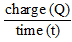or Q = IT ... (i)

Also, potential difference is the work done in bringing a charge Q from one end to the other end of the conductor. Thus

(Potential Difference)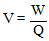W = VQ ... (ii)

From (i) and (ii), we get that

W = V(It)

W = VIT ... (iii)

But according to Ohm's law

V = IR ... (iv)

From equation (iii) and (iv), we get that

W = (IR)(IT)

W =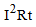But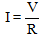and by putting this value in equation (iii), we get

W =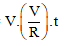W =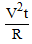This work done (W) by the current, measures the Electric Energy. Thus

W ∝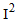W ∝ R

W ∝ t

Thus,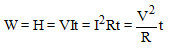(i) Commercial unit of electrical energy (Kilowatt - hour):

The S.I. unit of electrical energy is Joule and we know that for commercial purposes we use a bigger unit of electrical energy which is called "Kilowatt - hour". One Kilowatt - hour is the amount of electri-cal energy consumed when an electrical appliance having a power rating of 1 Kilowatt and is used for 1 Hour.

(ii) Relation between Kilowatt hour and Joule:

Kilowatt-hour is the energy supplied by a rate of working of 1000 watts for 1 hour.

1 kilowatt-hour= 3600000 joules

⇒ 1kWh=3.6 × 106J

### ELECTRIC POWER:

The rate at which electric energy is dissipated or consumed, is termed as electric power. The power P is given by, P = W/t = I2

The unit of electric power is watt, which is the power consumed when 1 A of current flows at a potential difference of 1V.

(i) Unit of power:

The S.i. unit of electric power is ‘watt' which is denoted by the letter W. The power of 1 watt is a rate of working of 1 joule per second.

A bigger unit of electric power is kilowatt.

1 kilowatt (kW) = 1000 watt.

Power of an agent is also expressed in horse power (hp).

1 hp = 746 watt.

(ii) Formula for calculating electric power:

We know, Power, P =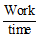And Work, W = V × I × t Joules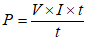P = V × I

P = V × I

Power P in terms of I and R :Now from Ohm's law we have,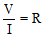V= I × R

P= I × R × I

P= I2 × R

Power P in terms of V and R: We know, P = V × I

From Ohm's law I =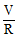P =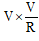P =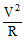### Power - Voltage Rating of Electrical Appliances:

Every electrical appliance like an electric bulb, radio or fan has a label or engraved plate on it which tells us the voltage (to be applied) and the electrical power consumed by it. For example, if we look at a particular bulb in our home, it may have the figures 220V, 100W written on it. Now, 220V means that this bulb is to be used on a voltage of 220 volts and 100W which means it has a power consumption of 100 watts or 100 joules per second.

### ELECTRIC FUSE:

An electric fuse is an easily fusible wire of short length put into an electrical circuit for protection purposes. It is arranged to melt ("blow") at a definite current. It is an alloy of lead and tin (37% lead + 63% tin). It has a low resistivity and low melting point. As soon as the safe limit of current exceeds, the fuse "blows" and the electric circuit is cut off.

Consider a wire of length L, radius r and resistivity ρ . Let I be the current flowing through the wire. Now rate at which heat is produced in the wire is,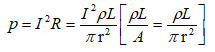This heat increases the temperature of the wire. Due to radiation some heat is lost. The temperature of the fuse becomes constant when the heat lost due to the radiation becomes equal to the heat produced due to the passage of current. This gives the value of current which can safely pass through the fuse.

In other words we have,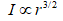Ex. 15 bulbs of 60W each, run for 6 hours daily and a refrigerator of 300 W runs for 5 hours daily. Work out per day bill at 3 rupees per unit.

Solution: Total wattage of 15 bulbs = 15 × 60W = 900W

∴ Electrical energy consumed by bulbs per day = P × t = 900 × 6 = 5400Wh

And electrical energy consumed by refrigerator per day = 300 × 5 = 1500 h

Total electrical energy consumed per day = (5400 + 1500)Wh = 6900Wh

∴ Electrical energy consumed per day =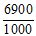KWh = 6.9 KWh

Here, per day bill = Rs. 6.9 × 3 = Rs. 20.7

Example: Two lamps, one rated 100W at 220V and other 60W at 220V are connected in parallel to a 220V supply. What is current drawn from the supply line?

Solution:  Given that V = 220V

P1 = 100W and P2 = 60W

∴ Current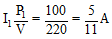Similarly,

Current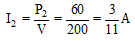Hence, total current drawn from the supply line =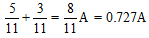.